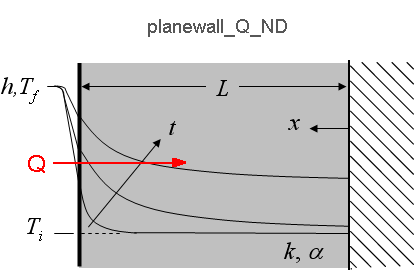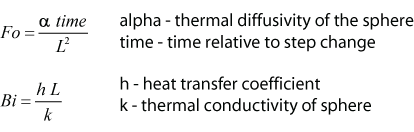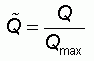Contents - Index

Planewall_Q_NDThe function planewall_Q_ND(Fo, Bi) returns the non-dimensional heat transfer to a bounded plane wall with one edge exposed to convection (x=L) and the other adiabatic (x=0). The supplied values, Fo, Bi represent the Fourier number and Biot number respectively.  The non-dimensional heat transfer is the ratio of the actual to the maximum heat transfer into the wall.  A separation of variables technique is used to solve for the temperature, as explained in section 3.5.2 of Nellis and Klein.

Inputs:Output:Example:
\$UnitSystem SI K Pa J
Fo=0.6
Bi=1.5
Q_hat=planewall_Q_ND(Fo, Bi)
Q_max=1000 [W/m^2]
Q=Q_max*Q_hat

{Solution:
Q=457.4 [W/m^2]
Q_hat=0.4574}

Transient Conduction Index